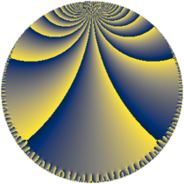# Properties

 Label 384.8.nLevel $384$ Weight $8$ Character orbit 384.n Rep. character $\chi_{384}(49,\cdot)$ Character field $\Q(\zeta_{8})$ Dimension $224$ Sturm bound $512$

# Related objects

## Defining parameters

 Level: $$N$$ $$=$$ $$384 = 2^{7} \cdot 3$$ Weight: $$k$$ $$=$$ $$8$$ Character orbit: $$[\chi]$$ $$=$$ 384.n (of order $$8$$ and degree $$4$$) Character conductor: $$\operatorname{cond}(\chi)$$ $$=$$ $$32$$ Character field: $$\Q(\zeta_{8})$$ Sturm bound: $$512$$

## Dimensions

The following table gives the dimensions of various subspaces of $$M_{8}(384, [\chi])$$.

Total New Old
Modular forms 1824 224 1600
Cusp forms 1760 224 1536
Eisenstein series 64 0 64

## Trace form

 $$224 q + O(q^{10})$$ $$224 q - 286832 q^{23} - 1429968 q^{31} + 1633008 q^{35} + 732368 q^{43} + 2993328 q^{51} + 3631264 q^{53} - 4191008 q^{55} - 3671872 q^{59} + 4559776 q^{61} + 4000752 q^{63} - 776272 q^{67} - 9580896 q^{69} - 24697408 q^{71} + 11260512 q^{75} + 23828896 q^{77} - 3406992 q^{91} + O(q^{100})$$

## Decomposition of $$S_{8}^{\mathrm{new}}(384, [\chi])$$ into newform subspaces

The newforms in this space have not yet been added to the LMFDB.

## Decomposition of $$S_{8}^{\mathrm{old}}(384, [\chi])$$ into lower level spaces

$$S_{8}^{\mathrm{old}}(384, [\chi]) \cong$$ $$S_{8}^{\mathrm{new}}(32, [\chi])$$$$^{\oplus 6}$$$$\oplus$$$$S_{8}^{\mathrm{new}}(96, [\chi])$$$$^{\oplus 3}$$$$\oplus$$$$S_{8}^{\mathrm{new}}(128, [\chi])$$$$^{\oplus 2}$$